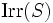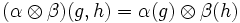# Outer tensor product establishes bijection between irreducible representations of direct factors and direct product

## Statement

Suppose$G$ and$H$ are groups and$k$ is a field. Let$\operatorname{Irr}(S)$ denote the set of irreducible representation of the group$S$ over$k$. Then, there is a natural bijection:$\operatorname{Irr}(G) \times \operatorname{Irr}(H) \leftrightarrow \operatorname{Irr}(G \times H)$.

The bijection is given using the outer tensor product of linear representations, as follows. For irreducible representations$\alpha,\beta$ of$G$ and$H$, to vector spaces$V$ and$W$,$\alpha \otimes \beta$ is defined as a linear representation on the tensor product$V \otimes W$, with:$(\alpha \otimes \beta)(g,h) = \alpha(g) \otimes \beta(h)$.